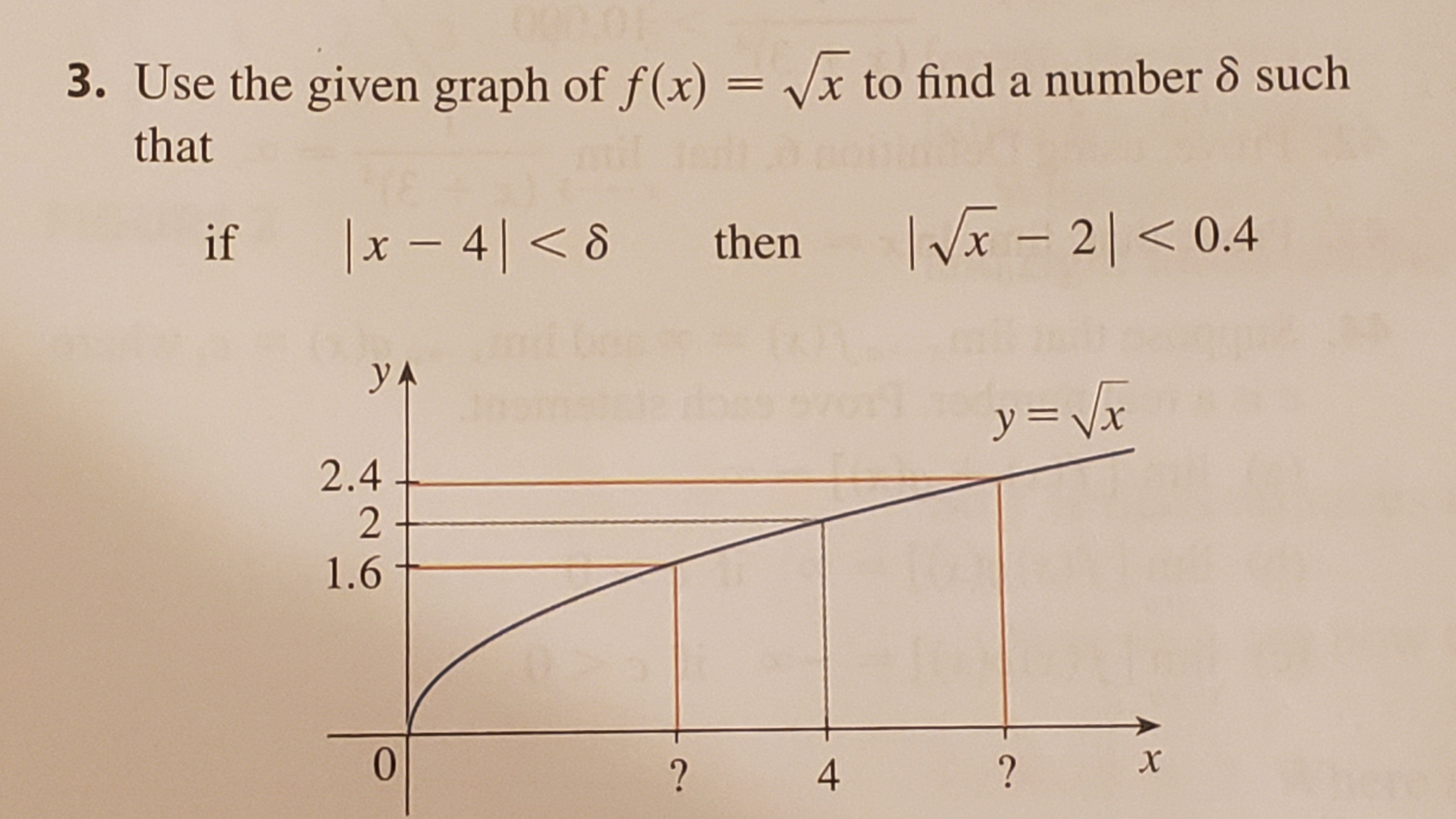# 3. Use the given graph of f(x) = vx to find a number 8 suchthatIVx - 20.4x48 thenifyAy Vr2.421.60x??4

Question
45 viewshelp_outlineImage Transcriptionclose3. Use the given graph of f(x) = vx to find a number 8 such that IVx - 20.4 x48 then if yA y Vr 2.4 2 1.6 0 x ? ? 4 fullscreen
check_circle

Step 1

Consider the second inequality.

Step 2

Using result, If | x-c | < a then -a < ( x-c ) < a. Simplify the above inequality as follows,

Step 3

Add two on all the three expressio...

### Want to see the full answer?

See Solution

#### Want to see this answer and more?

Solutions are written by subject experts who are available 24/7. Questions are typically answered within 1 hour.*

See Solution
*Response times may vary by subject and question.
Tagged in

### Calculus